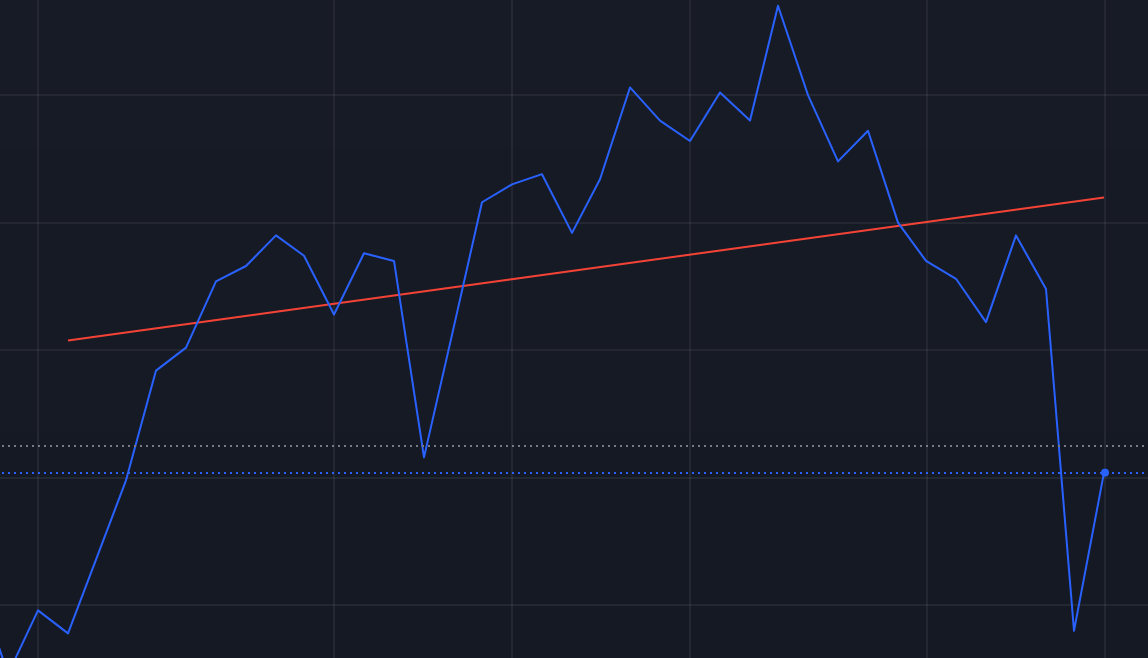# Euclidean Distance Matrix

Step by step explanation on how EDM is represented in linear algebra and how to code it as a function in Python in just one line.Pitagora : Euclide = Triangle : Geometry (drawing by : Andrea Grianti)

Hi everybody, in this post I want to explain my experience in figuring out how, a rather intuitive concept like that of the Euclidean…

# Cosine Similarity Matrix using broadcasting in Python

Learn how to code a (almost) one liner python function to calculate (manually) cosine similarity or correlation matrices used in many data science algorithms using the broadcasting feature of numpy library in Python.

Do you think we can say that a professional MotoGP rider and the kid in the picture…

# Linear Regression with TA-Lib

Understanding how the Linear Regression functions in TA-Lib Python LibraryThe Linear Regression functions within TA-Lib are a simplification of others Linear Regression functions available through other Python Libraries. Nevertheless it could be very useful in the context of Technical Analysis of stock market indicators (Source: Image of the author)

For those of you new to TA-Lib and Technical Analysis with Python, I have pasted in the following lines the introduction statement you can find in the website where the TA-Lib Python library is available for download.

So the quick…

# Feature Selection in PCA

My previous story was about the math intuition behind PCA. Today my objective is to apply those concepts to a simple use case and in particular to the part which often is overlooked being that of principal components interpretation.

As you probably know PCA is a sophisticated tool for dimensionality…

# How to: Sum, Mean, Variance, Covariance in Linear Algebra

If the title looks puzzling let me say that I believe that learning Python (or R) will take you to Machine Learning, but learning Linear Algebra will take you everywhere.

So going from using sw libraries to creating your stuff means moving with agility from numbers to vectors (and matrices)…

# K Means parameters and results (in R Studio) explained